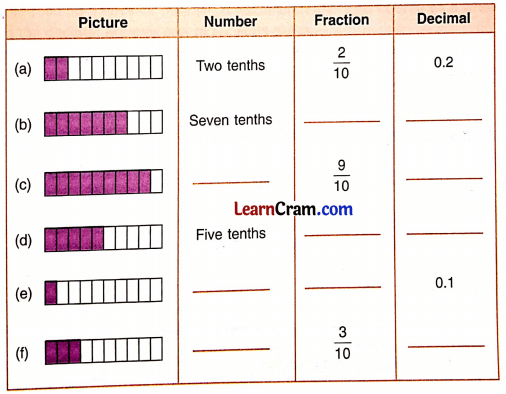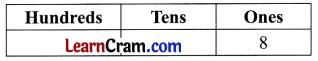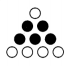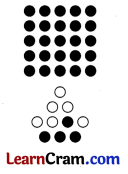# DAV Class 5 Maths Chapter 5 Worksheet 1 Solutions

The DAV Class 5 Maths Book Solutions Pdf and DAV Class 5 Maths Chapter 5 Worksheet 1 Solutions of Decimals offer comprehensive answers to textbook questions.

## DAV Class 5 Maths Ch 5 Worksheet 1 Solutions

Question 1.
Write as decimals.
(a) $$\frac{3}{10}$$
Solution:
$$\frac{3}{10}$$ = .3 or 0.3

(b) $$\frac{5}{10}$$
Solution:
$$\frac{5}{10}$$ = .5 or 0.5

(c) $$\frac{9}{10}$$
Solution:
$$\frac{9}{10}$$ = .9 or 0.9(d) $$\frac{8}{10}$$
Solution:
$$\frac{8}{10}$$ = .8 or 0.8

(e) $$\frac{7}{10}$$
Solution:
$$\frac{7}{10}$$ = .7 or 0.7

(f) $$\frac{1}{10}$$
Solution:
$$\frac{1}{10}$$ = .1 or 0.1

(g) $$\frac{6}{10}$$
Solution:
$$\frac{6}{10}$$ = .6 or 0.6

(h) $$\frac{4}{10}$$
Solution:
$$\frac{4}{10}$$ = .4 or 0.4Question 2.
Write as fractions.
(a) 0.2
Solution:
0.2 = $$\frac{2}{10}$$

(b) 0.4
Solution:
0.4 = $$\frac{4}{10}$$

(c) 0.7
Solution:
0.7 = $$\frac{7}{10}$$

(d) 0.6
Solution:
0.6 = $$\frac{6}{10}$$

(e) 0.5
Solution:
0.5 = $$\frac{5}{10}$$(f) 0.9
Solution:
0.9 = $$\frac{9}{10}$$

(g) 0.8
Solution:
0.8 = $$\frac{8}{10}$$

(h) 0.3
Solution:
0.3 = $$\frac{3}{10}$$

Question 3.
Read and write the number names. The first one is done for you.
(a) 7.5 ____________
Solution:
Seven and five tenths

(b) 9.4 ____________
Solution:
Nine and four tenths.

(c) 18.2 ____________
Solution:
Eighteen and two tenths.

(d) 65.8 ____________
Solution:
Sixty five and eight tenths.(e) 225.7 ____________
Solution:
Two hundred twenty five and seven tenths.

(f) 315.8 ____________
Solution:
Three hundred fifteen and eight tenths.

(g) 1238.7 ____________
Solution:
One thousand two hundred thirty eight and seven tenths.

(h) 2987.3 ____________
Solution:
Two thousand nine hundred eighty seven and three tenths.

(i) 1087.3 ____________
Solution:
One thousand eighty seven and three tenths.Question 4.
Write in decimal form. The first one is done for you.
(a) Eight tenths.
Solution:
0.8

(b) Five tenths.
Solution:
0.5

(c) Six and two tenths.
Solution:
6.2

(d) Fifty three and seven tenths.
Solution:
53.7

(e) Eighty two and three tenths.
Solution:
82.3

(f) One hundred thirty five and five tenths.
Solution:
135.5

(g) Five hundred eighty five and three tenths.
Solution:
585.3

(h) Two hundred twenty seven and seven tenths.
Solution:
227.7Question 5.
Fill in the blanks.Solution:DAV Class 5 Maths Chapter 5 Worksheet 1 Notes

Hindu Arabic System of Numeration: The system of numeration that we have studied is known as the Hindu Arabic System of Numeration.

International System of Numeration: A slight variation in number names is called the International System of Numeration.

Decimal System of Numeration: The system of numeration is known as the Decimal System of Numeration. The reason is that we use ten symbols – 1, 2, 3, 4, 5, 6, 7, 8, 9, 0 (digits) to write any number, howsoever large it may be.

Arrange number 8 in the place value chart:If we multiply 8 by 10, we get 80:If we divide 80 by 10, we get 8:So we observe when we multiply the number by 10 it jumps one column to the left. When we divide 8 tens by 10, 8 jumps one column to the right. Divide 8 again by 10. 8 will jump one more column to the right. We add one more column called tenths in the place value chart. Since $$\frac{8}{10}$$ is a fraction with a value less than 1, we separate by a point called the decimal point.
8 ÷ 10 = $$\frac{8}{10}$$ = .8 or 0.8
We read it as point 8 or decimal eight or zero decimal eight.Remember: Decimal takes less space in writing. It is easier to compare two fractional numbers using decimals.Example 1.
$$\frac{5}{10}$$
Solution:Number name – Five tenths
Fraction = $$\frac{5}{10}$$
Decimal = 0.5

Example 2.
25$$\frac{4}{10}$$
Solution:Number name – Twenty five and four tenths
Fraction – 25$$\frac{4}{10}$$
Decimal – 25.4